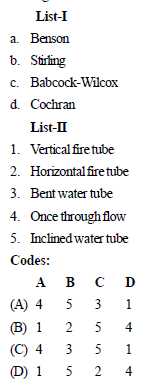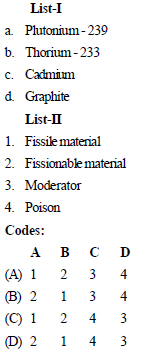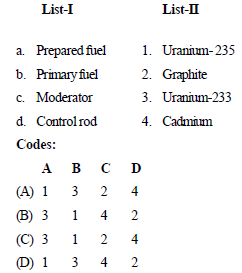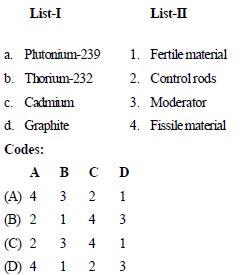Courses

# Test: Power Plant Level - 3

## 25 Questions MCQ Test Additional Documents & Tests for Mechanical Engineering | Test: Power Plant Level - 3

Description
This mock test of Test: Power Plant Level - 3 for Mechanical Engineering helps you for every Mechanical Engineering entrance exam. This contains 25 Multiple Choice Questions for Mechanical Engineering Test: Power Plant Level - 3 (mcq) to study with solutions a complete question bank. The solved questions answers in this Test: Power Plant Level - 3 quiz give you a good mix of easy questions and tough questions. Mechanical Engineering students definitely take this Test: Power Plant Level - 3 exercise for a better result in the exam. You can find other Test: Power Plant Level - 3 extra questions, long questions & short questions for Mechanical Engineering on EduRev as well by searching above.
QUESTION: 1

Solution:
QUESTION: 2

Solution:
QUESTION: 3

### Match List-I (Type of boiler) with List-II (Features) select the correct answer using the codes given below the lists:Solution:
QUESTION: 4

In a boiler, the air preheater is invariably located between:

Solution:
QUESTION: 5

Out of the following impurities generally found in water, which one requires special consideration in case of very high pressure boilers?

Solution:
QUESTION: 6

In a locomotive boiler, the draught is produced by

Solution:
QUESTION: 7

The function of economizer in a boiler is to

Solution:
QUESTION: 8

The pump preferred to be used for pumping highly viscous fluids belongs to the category of

Solution:
QUESTION: 9

When a hydrocarbon fuel burns in deficit air, Orsat analyzer will show the presence of following gases in the engine exhaust

Solution:
QUESTION: 10

The air pre-heater of a boiler is located between

Solution:
QUESTION: 11

A super critical boiler requires

Solution:
QUESTION: 12

Surging basically implies

Solution:
QUESTION: 13

Phenomenon of chocking in compressor means

Solution:
QUESTION: 14

The turbo machine used to circulate refrigerant in large refrigeration plant is

Solution:
QUESTION: 15

The energy transfer process is

Solution:
QUESTION: 16

Reciprocating compressors are provided with

Solution:

Explanation : Ring valves are typically used for slow speed, high pressure process compressors. Instead of individual poppets, these valves use concentric rings, which open and close the valve ports. Plate valves are typically used for high speed separable compressors.

QUESTION: 17

Stalling phenomena in an axial flow compressor stage is caused due to which one of the following?

Solution:
QUESTION: 18

In an axial flow compressor, stalling is the phenomenon of

Solution:
QUESTION: 19

Compressors used in gas turbines are of

Solution:
QUESTION: 20

Match List-I with List-II select the correct answer using the codes given below the lists:Solution:
QUESTION: 21

Match List-I with List-II select the correct answer using the codes given below the lists:Solution:
QUESTION: 22

Match List-I with List-II select the correct answer using the codes given below the lists:Solution:
QUESTION: 23

Moderator in a nuclear plant is used to

Solution:
QUESTION: 24

Enriched Uranium is one in which

Solution:
QUESTION: 25

The reflectivity in a reactor depends upon

Solution: